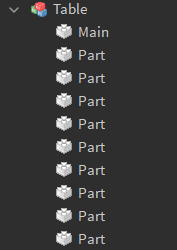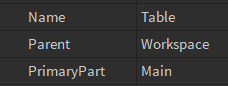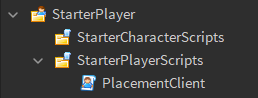# Starting a 3D Placement System

In this tutorial, I’ll guide you through starting a simple 3D placement system!

A few things to note:

• I won’t show you how to destroy objects and how to actually place them, in which case you’d use a RemoteEvent
• This will be a very bare-bones system meaning no special effects or features
• I will only go over pc support, but adding mobile or console support shouldn’t be too hard

## Setting up the model

First, you’ll need a model. You can always add some code to select models but for this tutorial we’ll be placing a simple table.

Your model needs to have a primary part which will be the centered and cover the entire model.
Warning: The primary part’s size needs to be a full number!
I made a new part named “Main” and set it to CanCollide false.Now, your model is fully set up!

## Setting up other stuff

Now, you want to add a LocalScript to StarterPlayerScripts## Client-side Programming

### Start

This system will use 3 keybinds:
E to start/stop building
R to rotate on the Y axis
T to turn on the Z axis

Let’s start by making a simple check for which key is pressed. Using a bunch of if statements isn’t the best solution but for now we’ll keep it as-is.

``````local userInputService = game:GetService("UserInputService")

local currentMode = nil -- We'll either set this to "Build" or nil
local currentObject = nil -- This will be the object being placed

userInputService.InputBegan:Connect(function(input, inGui)
if inGui then -- 2nd parameter is always true if the action happened in a gui
return
end

local key = input.KeyCode -- Here we get the Enum KeyCode which was pressed

if key == Enum.KeyCode.E then

elseif key == Enum.KeyCode.R then

elseif key == Enum.KeyCode.T then

end
end)
``````

### Toggling the mode

Great! Now our code checks which key was pressed. Let’s see how we can set the currentMode variable:

``````if currentObject then
currentObject:Destroy()
currentObject = nil
end

if currentMode ~= "Build" then
currentMode = "Build" -- Set currentMode to "Build"
currentObject = workspace.Table:Clone()
currentObject.Parent = workspace
else
currentMode = nil -- Otherwise, set it to nil, if we want to stop building
end
``````

Place this inside the if statement.

### Rotating

Now let’s quickly implement rotating and turning.

``````local orientation = CFrame.new()
``````

Now, here is how we can change the “orientation” variable when the player presses R

``````orientation = CFrame.Angles(0, math.rad(90), 0) * orientation -- CFrame.Angles uses radians so we need to convert 90 using math.rad
``````

…and T

``````orientation = CFrame.Angles(0, 0, math.rad(90)) * orientation-- CFrame.Angles uses radians so we need to convert 90 using math.rad
``````

Quite simple. Now place these in the respective if statements.

### Raycasting

Raycasting means casting an imaginary ray until it either hits something or becomes too long.
We can cast a ray from the mouse to see where the player’s mouse hit (in the world space) and use that when placing.

First, define these variables:

``````local mouseHitPart = nil
local mouseHitPos = nil
local mouseHitNormal = nil

local player = game:GetService("Players").LocalPlayer
local camera = workspace.CurrentCamera
``````

After that we write a simple raycast function like so:

``````local function raycast()
local mousePos = userInputService:GetMouseLocation() -- Returns a Vector2 of the mouse position

local params = RaycastParams.new()
params.FilterDescendantsInstances = {player.Character, currentObject} -- Ignore the object we're placing and the character
params.FilterType = Enum.RaycastFilterType.Blacklist

local unitRay = camera:ScreenPointToRay(mousePos.X, mousePos.Y) -- This creates a UnitRay from the mouse's position

return workspace:Raycast(unitRay.Origin, unitRay.Direction * 200, params)
end
``````

### Moving the model

First, we need a function to get the “rotated size” of a model.
This is because if a model has been rotated or turned, the X, Y, an Z size can’t be used as they don’t match up.

``````local function getRotatedSize(size)
local newModelSize = orientation * CFrame.new(size) -- Multiply the orientation by the size
newModelSize = Vector3.new(
math.abs(newModelSize.X),
math.abs(newModelSize.Y),
math.abs(newModelSize.Z)
) -- math.abs so our results aren't negative

return newModelSize
end
``````

Every Heartbeat (this event fires every frame) we check what the ray hit and set our 3 variables to that:

``````game:GetService("RunService").Heartbeat:Connect(function()
local raycastResult = raycast() or {}

mouseHitPart = raycastResult.Instance
mouseHitPos = raycastResult.Position
mouseHitNormal = raycastResult.Normal
end)

``````

Now comes one of the most important functions - we need to get a snapped position for where to place the model:

``````local function getPlacementPos(size)
local newModelSize = getRotatedSize(size)

return Vector3.new(
math.floor(mouseHitPos.X / 0.5 + 0.5) * 0.5 + mouseHitNormal.X * (newModelSize.X / 2),
math.floor(mouseHitPos.Y / 0.5 + 0.5) * 0.5 + mouseHitNormal.Y * (newModelSize.Y / 2),
math.floor(mouseHitPos.Z / 0.5 + 0.5) * 0.5 + mouseHitNormal.Z * (newModelSize.Z / 2)
) -- Snap the position to 0.5 and offset it using the surface normal
end
``````

Finally, every heartbeat, update the variables and position the model:

``````game:GetService("RunService").Heartbeat:Connect(function()
local raycastResult = raycast() or {} -- Get the returned raycast result

mouseHitPart = raycastResult.Instance
mouseHitPos = raycastResult.Position
mouseHitNormal = raycastResult.Normal

if currentMode == "Build" then
if mouseHitNormal and mouseHitPos and currentObject then
currentObject:SetPrimaryPartCFrame( -- Set the primary part cframe
CFrame.new(getPlacementPos(currentObject.PrimaryPart.Size)) -- Snapped position
* orientation -- Multiplied by orientation
)
end
end
end)
``````

## Finishing up

Your final code should look something like this:

Code
``````local userInputService = game:GetService("UserInputService")

local orientation = CFrame.new()

local currentMode = nil
local currentObject = nil
local mouseHitPart = nil
local mouseHitPos = nil
local mouseHitNormal = nil

local player = game:GetService("Players").LocalPlayer
local camera = workspace.CurrentCamera

userInputService.InputBegan:Connect(function(input, inGui)
if inGui then
return
end

local key = input.KeyCode

if key == Enum.KeyCode.E then
if currentObject then
currentObject:Destroy()
currentObject = nil
end

if currentMode ~= "Build" then
currentMode = "Build"

currentObject = workspace.Table:Clone()
currentObject.Parent = workspace
else
currentMode = nil
end
elseif key == Enum.KeyCode.R then
orientation = CFrame.Angles(0, math.rad(90), 0) * orientation
elseif key == Enum.KeyCode.T then
orientation = CFrame.Angles(0, 0, math.rad(90)) * orientation
end
end)

local function raycast()
local mousePos = userInputService:GetMouseLocation()

local params = RaycastParams.new()
params.FilterDescendantsInstances = {player.Character, currentObject}
params.FilterType = Enum.RaycastFilterType.Blacklist

local unitRay = camera:ScreenPointToRay(mousePos.X, mousePos.Y)

return workspace:Raycast(unitRay.Origin, unitRay.Direction * 200, params)
end

local function getRotatedSize(size)
local newModelSize = orientation * CFrame.new(size)
newModelSize = Vector3.new(
math.abs(newModelSize.X),
math.abs(newModelSize.Y),
math.abs(newModelSize.Z)
)

return newModelSize
end

local function getPlacementPos(size)
local newModelSize = getRotatedSize(size)

return Vector3.new(
math.floor(mouseHitPos.X / 0.5 + 0.5) * 0.5 + mouseHitNormal.X * (newModelSize.X / 2),
math.floor(mouseHitPos.Y / 0.5 + 0.5) * 0.5 + mouseHitNormal.Y * (newModelSize.Y / 2),
math.floor(mouseHitPos.Z / 0.5 + 0.5) * 0.5 + mouseHitNormal.Z * (newModelSize.Z / 2)
)
end

game:GetService("RunService").Heartbeat:Connect(function()
local raycastResult = raycast() or {}

mouseHitPart = raycastResult.Instance
mouseHitPos = raycastResult.Position
mouseHitNormal = raycastResult.Normal

if currentMode == "Build" then
if mouseHitNormal and mouseHitPos and currentObject then
currentObject:SetPrimaryPartCFrame(
CFrame.new(getPlacementPos(currentObject.PrimaryPart.Size))
* orientation
)
end
end
end)
``````

Once you have that, you can now move your model around on a fixed 0.5 grid!

I might make a part 2 where I cover actually placing the objects on the server and deleting objects as well.

If you have any feedback on this tutorial let me know how I can improve! Thanks for reading!

48 Likes

Great tutorial! Perfect for those who are learning how placement system’s work as well, well done

4 Likes

I’ve been looking for something like this for a very long time. Thanks!2 Likes

I would love a part 2 thank you

3 Likes

You can use InputChanged instead of the Heartbeat event. This is more optimized since you could just raycast only when they move the mouse.

Here’s an example of how to replace the Heartbeat connection:

``````local uis = game:GetService("UserInputService")
uis.InputChanged:Connect(function(input, processed)
-- not processed = (mouse not on ui)
if not processed and input.UserInputType == Enum.UserInputType.MouseMovement then
local raycastResult = raycast()
if currentMode == "Build" and raycastResult then
mouseHitPart = raycastResult.Instance
mouseHitPos = raycastResult.Position
mouseHitNormal = raycastResult.Normal
currentObject:SetPrimaryPartCFrame(
CFrame.new(getPlacementPos(currentObject.PrimaryPart.Size))
* orientation
)
end
end
end)
``````

EDIT: `RaycastResult` behavior differs from last time I remembered. It can now be `nil` which I assume is the case when an Instance isn’t found. Code has been updated.

5 Likes

Thanks for the feedback! I’ll update the tutorial with your suggestions soon.

2 Likes